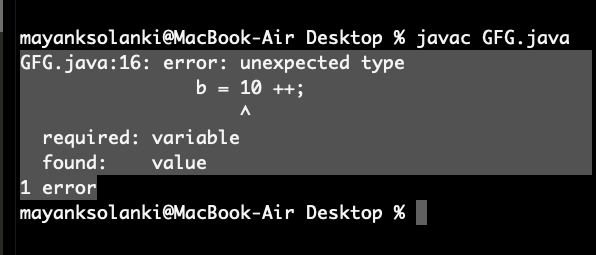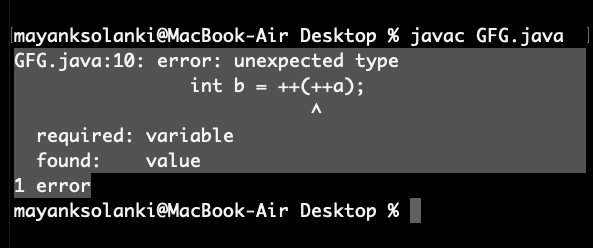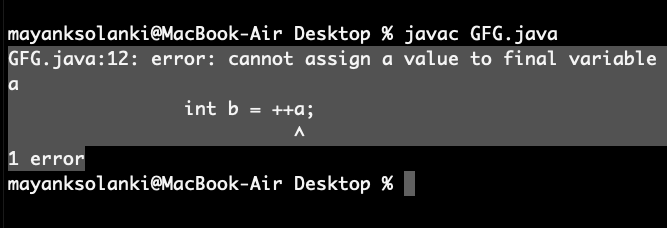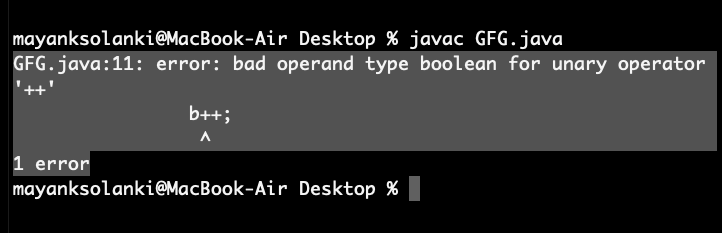Open In App

# Interesting facts about Increment and Decrement operators in Java

Increment operator is used to increment a value by 1. There are two varieties of increment operator:

• Post-Increment: Value is first used for computing the result and then incremented.
• Pre-Increment: Value is incremented first and then the result is computed.

Example

## Java

 `//INCREMENTAL OPERATOR``//Let's see what exactly happens when we use these operators with the help of a code.` `import` `java.io.*;` `class` `GFG {``    ``public` `static` `void` `main (String[] args) {``        ``//1. Post-Increment Operator``      ``int` `a = ``5``;``      ``int` `b = ``7``;``      ` `      ``int` `c = a++ + b; ``//Here 'a' will not incremented immediately, a++ will still return value 5.``      ``//  c = 5   + 7 and this will evaluate to 12.``      ` `      ``System.out.println(``"Post- Increment \n c = "``+ c);``      ` `      ``//2. Pre-Increment Operator``      ` `      ``int` `A = ``5``;``      ``int` `B = ``7``;``      ` `      ``int` `C = ++A + B; ``//Here 'a' will be incremented immediately, and ++a will return value 6.``      ``//  C =  6  + 7   and this will evaluate to 13.``      ` `      ``System.out.println(``"Pre- Increment \n C = "``+ C);``     ` `      ``//Example-``      ``int` `m = ``1``, n = ``2``;``      ` `      ``int` `o = m++ + n + ++m; ``// It goes like m++ = 1, n = 2, ++m = 1+ incremented 'm' from m++``      ``//                                                         = 1 + (1+m) = 1+ (1+1) = 3``      ``//  o = 1   + 2 +   3 = 6 ``      ``System.out.println(``"Example \n o = "``+ o);``    ``}``}`

Decrement operator is used for decrementing the value by 1. There are two varieties of decrement operators.

• Post-decrement: Value is first used for computing the result and then decremented.
• Pre-decrement: Value is decremented first and then the result is computed.

Example

## Java

 `//DECREMETAL OPERATORS``//Let's see what exactly happens when we use these operators with the help of a code.``import` `java.io.*;` `class` `GFG {``    ``public` `static` `void` `main (String[] args) {``         ``//1. Post-Decrement Operator``      ``int` `a = ``5``;``      ``int` `b = ``7``;``      ` `      ``int` `c = a-- + b; ``//Here 'a' will not decremented immediately, a-- will still return value 5.``      ``//  c = 5   + 7 and this will evaluate to 12.``      ` `      ``System.out.println(``"Post- Decrement \n c = "``+ c);``      ` `      ``//2. Pre-Decrement Operator``      ` `      ``int` `A = ``5``;``      ``int` `B = ``7``;``      ` `      ``int` `C = --A + B; ``//Here 'a' will be decremented immediately, and --a will return value 4.``      ``//  C =  4  + 7   and this will evaluate to 11.``      ` `      ``System.out.println(``"Pre- Decrement \n C = "``+ C);``     ` `      ``//Example-``      ``int` `m = ``3``, n = ``2``;``      ` `      ``int` `o = m-- + n + --m; ``// It goes like m-- = 3, n = 2, --m = (decremented 'm' from 'm--') - 1;``      ``//                                                         = (m -1) - 1 = (3 -1) -1 = 1``      ``//  o = 3   + 2 +   1 = 6 ``      ``System.out.println(``"Example \n o = "``+ o);``    ``}``}`

Now let us do Interesting facts about Increment and Decrement operators:

• Can only be applied to variables only
• Nesting of both operators is not allowed
• They are not operated over final variables
• Increment and Decrement Operators can not be applied to boolean.

Let us discuss these 4 facts as listed above and do implement them as follows:

Fact 1: Can be applied to variables only

We can apply ++ and — operator only for variables but not for the constant values. If we are trying to apply ++ and — operator on the constant value then we will get a compile-time error which we will be seeing in example 1B after the below example as follows:

Example 1:

## Java

 `// Java program to illustrate Increment``// and Decrement Operators``// Can be Applied to Variables Only` `// Main class``public` `class` `GFG {``  ` `    ``// main driver method``    ``public` `static` `void` `main(String[] args)``    ``{``      ` `        ``int` `a = ``10``;``        ``int` `b = ++a;``       ` `       ``// Printing value inside variable``        ``System.out.println(b);``    ``}``}`

Output

`11`

Example 2:

## Java

 `// Java program to Illustrate Increment and Decrement``// operators Can be applied to variables only` `// Main class``public` `class` `GFG {` `    ``// main driver method``    ``public` `static` `void` `main(String[] args)``    ``{``        ``// Declaring and initializing variable``        ``int` `a = ``10``;` `        ``int` `b = ++a;` `        ``// This is change made in above program``        ``// which reflects error during compilation``        ``b = ``10` `++;` `        ``// Printing its value``        ``System.out.println(b);``    ``}``}`

Output:Fact 2: Nesting of both ++ and — operators are not allowed

Example

## Java

 `// Java program to Illustrate Nesting Can Not be Applied``// to Increment and Decrement Operators` `// Main class``public` `class` `GFG {` `    ``// Main driver method``    ``public` `static` `void` `main(String[] args)``    ``{``        ``int` `a = ``10``;``        ``int` `b = ++(++a);` `        ``// Printing the value inside variable``        ``System.out.println(b);``    ``}``}`

Output:Fact 3: Final variables can’t apply increment and decrement operator

The increment and decrement operators can not be applied to final variables because of the simple reason that their value can not be changed.

Example

## Java

 `// Java Program to Illustrate Increment and Decrement``// Operators Can not be applied to final variables` `// Main class``public` `class` `GFG {` `    ``// Main driver method``    ``public` `static` `void` `main(String[] args)``    ``{``        ``// Declaring and initializing final variable``        ``final` `int` `a = ``10``;` `        ``int` `b = ++a;` `        ``// Trying to print the updated value inside variable``        ``System.out.println(b);``    ``}``}`

Output:Fact 4: Increment and Decrement Operators can not be applied to boolean

We can apply ++ and — operators for all primitive data types except Boolean type as it only has true and false which even sounds impractical.

Example

## Java

 `// Java program to Illustrate Increment and Decrement``// Operators Can not be applied boolean data type` `// Main class``public` `class` `GFG {` `    ``// Main driver method``    ``public` `static` `void` `main(String[] args)``    ``{``        ``// Initially declaring boolean as false``        ``boolean` `b = ``false``;``        ``b++;` `        ``// Trying printing the bool value``        ``System.out.println(b);``    ``}``}`

Output:This article is contributed by Bishal Kumar Dubey. If you like GeeksforGeeks and would like to contribute, you can also write an article using write.geeksforgeeks.org or mail your article to review-team@geeksforgeeks.org. See your article appearing on the GeeksforGeeks main page and help other Geeks.